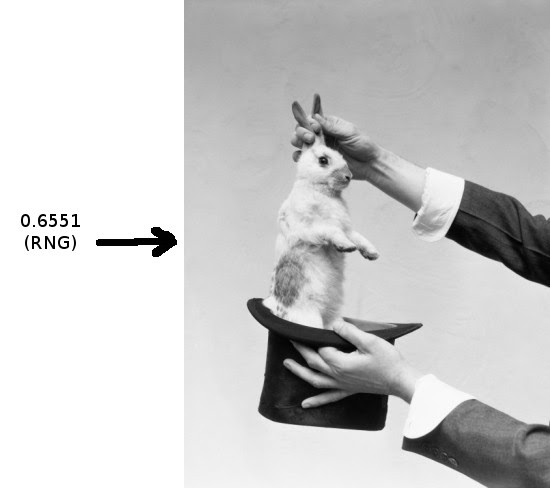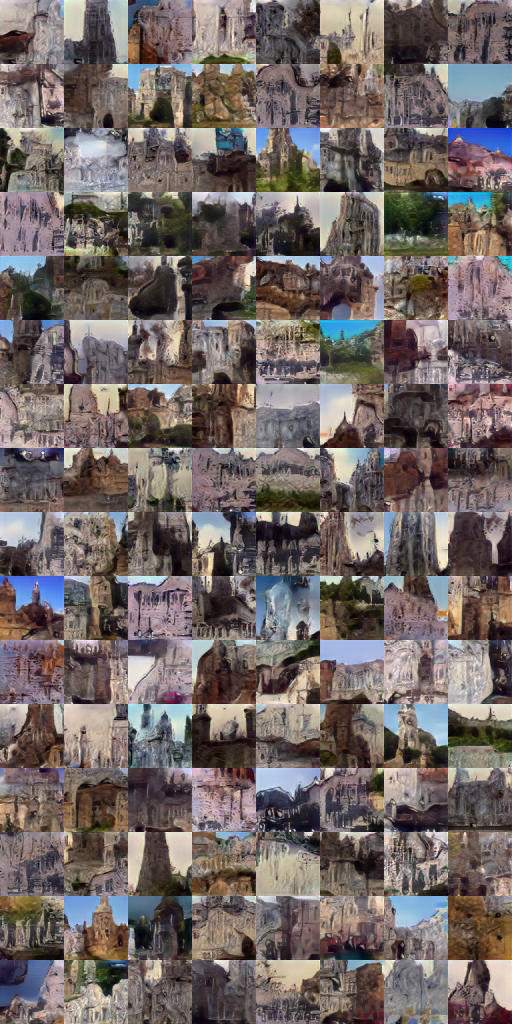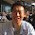## Saturday, June 25, 2016

### Generative Adversarial Nets in TensorFlow (Part I)

This post was first published on 12/29/15, and has since been migrated to Blogger.

This is a tutorial on implementing Ian Goodfellow's Generative Adversarial Nets paper in TensorFlow. Adversarial Nets are a fun little Deep Learning exercise that can be done in ~80 lines of Python code, and exposes you (the reader) to an active area of deep learning research (as of 2015): Generative Modeling!

Code on Github

### Scenario: Counterfeit Money

To help explain the motivations behind the paper, here's a hypothetical scenario:
Danielle is a teller at a bank, and one of her job responsibilities is to discriminate between real money and counterfeit money. George is a crook and is trying to make some counterfeit money, becase free money would be pretty radical.

Let's simplify things a bit and assume the only distinguishing feature of currency is one unique number, $X$, printed on the each bill. These numbers are randomly sampled from a probability distribution, whose density function $p_{data}$ is only known to the Treasury (i.e. neither Danielle nor George know the function). For convenience, this tutorial uses $p_{data}$ to refer to both the distribution and the density function (though semantically a distribution and its density function are not the same).

George's goal is to generate samples $x^\prime$ from $p_{data}$, so his counterfeit currency is indistinguishable from "real" currency. You might ask: how can George generate samples from $p_{data}$ if he doesn't know $p_{data}$ in the first place?

We can create computationally indistinguishable samples without understanding the "true" underlying generative process . The underlying generative process is the method that the Treasury itself is using to generate samples of $X$ - perhaps some efficient algorithm for sampling $p_{data}$ that relies on the analytical formula for the pdf.

We can think of this algorithm as the "natural (function) basis", the straightforward method the Treasury would actually use to print our hypothetical currency. However, a (continuous) function can be represented as a combination of a different set of basis functions; George can express the same sampler algorithm in a "neural network basis" or "Fourier basis" or other basis that can be used to build a universal approximator. From an outsider's perspective, the samplers are computationally indistinguishable, and yet George's model doesn't reveal to him the structure of the "natural" sampler basis or the analytical formula of $p_{data}$.

### Background: Discriminative vs. Generative Models

Let $X$, $Y$ be the "observed" and "target" random variables. The joint distribution for $X$ and $Y$ is $P(X,Y)$, which we can think of as a probability density over 2 (possibly dependent) variables.

A Discriminative model allows us to evaluate the conditional probability $P(Y|X)$. For instance, given a vector of pixel values $x$, what is the probability that $Y=6$? (where "6" corresponds to the categorical class label for "tabby cat"). MNIST LeNet, AlexNet, and other classifiers are examples of a discriminative models.On the other hand, a Generative model can allows us to evaluate the joint probability $P(X,Y)$. This means that we can propose value pairs $(X,Y)$ and do rejection-sampling to obtain samples $x$,$y$ from $P(X,Y)$. Another way to put this is that with the right generative model, we can convert a random number from $[0,1]$ into a picture of a rabbit. That's awesome.Of course, generative models are much harder to construct than discriminative models, and both are active areas of research in statistics and machine learning.

Goodfellow's paper proposes a very elegant way to teach neural networks a generative model for any (continuous) probability density function. We build two neural networks $D$ (Danielle) and $G$ (George), and have them play an adversarial cat-and-mouse game: $G$ is a generator and attempts to counterfeit samples from $p_{data}$ and $D$ is a decider that tries to not get fooled. We train them simultaneously, so that they both improve by competing with each other. At convergence, we hope that $G$ has learned to sample exactly from $p_{data}$, at which point $D(x)=0.5$ (random guessing).

Advesarial Nets have been used to great success to synthesize the following from thin air:Cat FacesChurchesAnime Characters

In this tutorial we won't be doing anything nearly as amazing, but hopefully you'll come away with a better fundamental understanding of adversarial nets.

### Implementation

We'll be training a neural network to sample from the simple 1-D normal distribution $\mathcal{N}(-1,1)$Let $D$,$G$ be small 3-layer perceptrons, each with a meager 11 hidden units in total. $G$ takes as input a single sample of a noise distribution: $z \sim \text{uniform}(0,1)$. We want $G$ to map points $z_1,z_2,...z_M$ to $x_1,x_2,...x_M$, in such a way that mapped points $x_i=G(z_i)$ cluster densely where $p_{data}(X)$ is dense. Thus, G takes in $z$ and generates fake data $x^\prime$.Meanwhile, the discriminator $D$, takes in input $x$ and outputs a likelihood of the input belonging to $p_{data}$.
Let $D_1$ and $D_2$ be copies of $D$ (they share the same parameters so $D_1(x)=D_2(x)$). The input to $D_1$ is a single sample of the legitimate data distribution: $x \sim p_{data}$, so when optimizing the decider we want the quantity $D_1(x)$ to be maximized. $D_2$ takes as input $x^\prime$ (the fake data generated by $G$), so when optimizing $D$ we want to $D_2(x^\prime)$ to be minimized. The value function for $D$ is:
$$\log(D_1(x))+\log(1-D_2(G(z)))$$
Here's the Python code:
batch=tf.Variable(0)
obj_d=tf.reduce_mean(tf.log(D1)+tf.log(1-D2))
.minimize(1-obj_d,global_step=batch,var_list=theta_d)

The reason we go through the trouble of specifying copies $D_1$ and $D_2$ is that in TensorFlow, we need one copy of $D$ to process the input $x$ and another copy to process the input $G(z)$; the same section of the computational graph can't be re-used for different inputs.
When optimizing $G$, we want the quantity $D_2(X^\prime)$ to be maximized (successfully fooling $D$). The value function for $G$ is:
$$\log(D_2(G(z)))$$
batch=tf.Variable(0)
obj_g=tf.reduce_mean(tf.log(D2))
.minimize(1-obj_g,global_step=batch,var_list=theta_g)

Instead of optimizing with one pair $(x,z)$ at a time, we update the gradient based on the average of $M$ loss gradients computed for $M$ different $(x,z)$ pairs. The stochastic gradient estimated from a minibatch is closer to the true gradient across the training data.
The training loop is straightforward:
# Algorithm 1, GoodFellow et al. 2014
for i in range(TRAIN_ITERS):
x= np.random.normal(mu,sigma,M) # sample minibatch from p_data
z= np.random.random(M)  # sample minibatch from noise prior
sess.run(opt_d, {x_node: x, z_node: z}) # update discriminator D
z= np.random.random(M) # sample noise prior
sess.run(opt_g, {z_node: z}) # update generator G


#### Manifold Alignment

Following the above recipe naively will not lead to good results, because we are sampling $p_{data}$ and $\text{uniform}(0,1)$ independently each iteration. Nothing is enforcing that adjacent points in the $Z$ domain are being mapped to adjacent points in the $X$ domain; in one minibatch, we might train $G$ to map $0.501 \to -1.1$, $0.502 \to 0.01$, and $0.503 \to -1.11$. The mapping arrows cross each other too much, making the transformation very bumpy. What's worse, the next minibatch might map $0.5015 \to 1.1$, $0.5025 \to -1.1$, and $0.504 \to 1.01$. This implies a completely different mapping $G$ from the previous minibatch, so the optimizer will fail to converge.To remedy this, we want to minimize the total length of the arrows taking points from $Z$ to $X$, because this will make the transformation as smooth as possible and easier to learn. Another way of saying this is that the "vector bundles" carrying $Z$ to $X$ should be correlated between minibatches.

First, we'll stretch the domain of $Z$ to the same size of $X$. The normal distribution centered at -1 has most of its probability mass lying between $[-5,5]$, so we should sample $Z$ from $\text{uniform}(-5,5)$. Doing this means $G$ no longer needs to learn how to "stretch" the domain $[0,1]$ by a factor of 10. The less $G$ has to learn, the better.
Next, we'll align the samples of $Z$ and $X$ within a minibatch by sorting them both from lowest to highest.

Instead of sampling $Z$ via np.random.random(M).sort(), we'll use via stratified sampling - we generate $M$ equally spaced points along the domain and then jitter the points randomly. This preserves sorted order and also increases the representativeness the entire training space. We then match our stratified, sorted $Z$ samples to our sorted $X$ samples.Of course, for higher dimensional problems it's not so straightforward to align the input space $Z$ with the target space $X$, since sorting points doesn't really make sense in 2D and higher. However, the notion of minimizing the transformation distance between the $Z$ and $X$ manifolds still holds .

The modified algorithm is as follows:
for i in range(TRAIN_ITERS):
x= np.random.normal(mu,sigma,M).sort()
z= np.linspace(-5.,5.,M)+np.random.random(M)*.01 # stratified
sess.run(opt_d, {x_node: x, z_node: z})
z= np.linspace(-5.,5.,M)+np.random.random(M)*.01
sess.run(opt_g, {z_node: z})


This step was crucial for me to get this example working: when dealing with random noise as input, failing to align the transformation map properly will give rise to a host of other problems, like massive gradients that kill ReLU units early on, plateaus in the objective function, or performance not scaling with minibatch size.

#### Pretraining D

The original algorithm runs $k$ steps of gradient descent on $D$ for every step of gradient descent on $G$. I found it more helpful to pre-train $D$ a larger number of steps prior to running the adversarial net, using a mean-square error (MSE) loss function to fit $D$ to $p_{data}$. This loss function is nicer to optimize than the log-likelihood objective function for $D$ (since the latter has to deal with stuff from $G$). It's easy to see that $p_{data}$ is the optimal likelihood decision surface for its own distribution.

Here is the decision boundary at initialization.After pretraining:Close enough, lol.

• Using too many parameters in the model often leads to overfitting, but in my case making the network too big failed to even converge under the minimax objective - the network units saturated too quickly from large gradients. Start with a shallow, tiny network and only add extra units/layers if you feel that the size increase is absolutely necessary.
• I started out using ReLU units but the units kept saturating (possibly due to manifold alignment issues). The Tanh activation function seemed to work better.
• I also had to tweak the learning rate a bit to get good results.

### Results

Before training, here is $p_{data}$, the pre-trained decision surface of $D$, and the generative distribution $p_g$.
.Here's the loss function as a function of training iterations.After training, $p_g$ approximates $p_{data}$, and the discriminator is about uniformly confused ($D=0.5$) for all values of $X$.And there you have it! $G$ has learned how to sample (approximately) from $p_{data}$ to the extent that $D$ can no longer separate the "reals" from the forgeries.

### Footnotes

1. Here's a more vivid example of computational indistinguishability: suppose we train a super-powerful neural network to sample from the distribution of cat faces.
The underlying (generative) data distribution for "true" cat faces involves 1) a cat being born and 2) somebody eventually taking a photograph of the cat. Clearly, our neural network isn't learning this particular generative process, as no real cats are involved. However, if our neural network can generate pictures that we cannot distinguish from "authentic" cat photos (armed with polynomial-time computational resources), then in some ways these photos are "just as legitimate" as regular cat photos.
This is worth pondering over, in the context of the Turing Test, Cryptography, and fake Rolexes.
2. Excessive mapping crossover can be viewed from the perspective of "overfitting", where the learned regression/classification function has been distorted in "contradictory" ways by data samples (for instance, a picture of a cat classified as a dog). Regularization techniques implicitly discourage excessive "mapping crossover", but do not explicitly implement a sorting algorithm to ensure the learned transformation is continuous / aligned in $X$-space with respect to $Z$-space. Such a sorting mechanism might be very useful for improving training speeds...

1.Awesome! Thank you for a well explained article!

2.Thanks for the post!

But I wonder what do you mean by "Another way to put this is that with the right generative model, we can convert a random number from [0,1] into a picture of a rabbit." ?

1.The inverse transform method converts a sample of Uniform(0,1) distribution into a sample of any other distribution (as long as the cumulative density function is invertible). There exists some function that maps (a sample from 0-1) to (a sample from the distribution of rabbit images). Such a function is highly complex and likely has no analytical formula, but a neural network can learn to approximate such a function.

The article mentions rejection sampling, which is a different technique that does not directly map from [0,1] to the sample of the target distribution. Thanks for helping me clarify this!

3.What was citation  supposed to be? Thx

4.5.You proposed 'centered at -1 has most of its probability mass lying between [−5,5]'
But...
Why not 'is [−6,4]'??

1.because mean is zero

2.The mean is -1. I think [-5,5] was chosen somewhat arbitrarily. Remember that G does not know what the distribution is or what the mean is.

6.7.Nice post, Thanks

8.Thanks for the post! I have one question: why does the outcome of training depend on the order the training samples (or whether x and z matches)?

1.I have the same question!

9.This comment has been removed by a blog administrator.

10.Great post, one short question. As far as I understood, it seems that the optimizer does not have to be subtracted from 1; e.g. opt_g=tf.~.minimize(1-obj_g,~) -> opt_g=tf.~.minimize(-obj_g,~), same for opt_d. Am I right? Is there any reason you have specified it to be 1? I am confused because, in the original paper, I could not find any mention about the 1 you have subtracted the objective functions from.

11.Hi everyone,
I would like to ask a question about dcgan. When i try to generate a single image from a single z vector using generative model of dcgan, it produces an average image but i try to generate a batch of images from a batch of z vectors, it produces reasonable results. How can we generate a single image from a single z vector?

12.This comment has been removed by the author.

13.Thank you for this enlightening post. I am trying to rebuild this example in R but I have difficulties.

I posted the following question: http://stackoverflow.com/questions/41783117/building-a-simple-generative-adversarial-net-with-neuralnet-in-r

Even if you don't know R the sourcecode should be clear enough so perhaps you can help? Any hint is highly appreciated - Thank you!

14.Thank you for the explanation. I'm doing some test with a similar code to the one that is in github because I need it for a project and I have a question:
In the multilayer preceptron, why you choose the second dimension of the w1 as 6, and then the w2 dimensions as (6,5). Does it has something to do with the normal distribution? I'm doing test with other dimensions and if they are not too big the results are quite similar.
Can anybody explain me why these dimensions are chosen? and why if I put much bigger dimensions the results are so bad?

Thank you so much!

15.Nice info Thanks for sharing it
Python Training in Chennai

16.Thanks for posting useful information.You have provided an nice article, Thank you very much for this one. And i hope this will be useful for many people.. and i am waiting for your next post keep on updating these kinds of knowledgeable things...Really it was an awesome article...very interesting to read..
Android training in chennai
Ios training in chennai

17.It's interesting that many of the bloggers your tips helped to clarify a few things for me as well as giving.. very specific nice content. And tell people specific ways to live their lives.Sometimes you just have to yell at people and give them a good shake to get your point across.
Web Design Company
Web Development Company
Mobile App Development Company

18.Hi,

19.